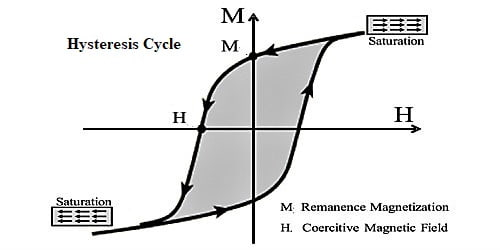# Cycle of Magnetization and Hysteresis

The cycle of Magnetization and Hysteresis

Out of the three types of magnetic materials only ferromagnetic materials have hysteresis property. By inserting a ferromagnetic material like iron inside a solenoid, the current is allowed to change slowly in the solenoid coil, and then I-H curve can be obtained.H versus I graph is called I-H graph. The graph is shown in Figure. We know that value of the intensity of magnetization I depend on the magma field intensity H. This is because that ‘I’ is created due to H. If the value of field intensity is charged in cyclic order an unusual behavior is observed which is shown in the figure. Scientist J. A. Euwong invented this phenomenon after many experiments. The graph of H versus I is called I-H graph. Magnetization curve is the relationship between altering the magnetic field and its pressure on the magnetic flux density. This curve has three regions. This is discussed below.

Let a ferromagnetic substance is placed in a magnetic field intensity H and lets it be magnetized. With the change of H intensity of magnetization, I also change and ‘I’ versus ‘H’ is represented by the curve “O a b c d e f g b”. The curve has been drawn by placing H along the X-axis and ‘I’ along the Y-axis. While H increases from zero intensity of magnetization ‘I’ start increasing. This is shown by the line ‘Oab’. After reaching the value of ‘I’ at ‘b’, a further increase of ‘I’ does not take place even if ‘H’ is increased. In this condition intensity of magnetization becomes saturated.

At saturation the value of ‘I’ is ‘OC’ and that of ‘H’ is ‘OA’. Under this situation, magnetic molecules of the magnetic substance remain aligned along a particular line.

Now, if the value of ‘H’ is decreased ‘I’ also start decreasing. But it does not come back to the initial point ‘O’. Then it follows the path ‘bc’. At point ‘c’ the value of ‘H’ becomes zero but ‘I’ is not zero. In this situation, some value of ‘I’ remains. It is shown by ‘Oc’ in the figure. This value of ‘I’ is called remanence and the ability of the magnetic material is called retentivity.

Now, by changing H in the opposite direction and if its negative values are increased then the Alice of ‘I’ gradually decreases which is shown by the line ‘cd’. At point,’d’ the value ‘I’ becomes zero. In this state ‘H’ is shown by ‘ad’. The value of ‘H’ when ‘I’ is zero is called coercive force and this property of the magnetic substance is called coercivity. In this condition, the magnetic substance loses its magnetism. If the value of H is allowed to increase in the negative direction the value of ‘I’ also becomes negative. It is shown by line de. But at one stage I acquire negative saturation value. In this condition I = OE and H = OD. In the figure point, ‘e’ represents this condition. Now if the negative value of H is increased further, the value of ‘I’ does not increase.

Again, if the value of ‘H’ is increased slowly in the positive direction, the value of ‘I’ also increases and following the path ‘efgb’ it reaches to the point ‘b’. After reaching ‘b’, it acquires the previous saturated value.

So, it is seen that the value of ‘I’ lags behind the value of H. It never becomes equal to or leads the value of H. This lagging of ‘I’ is called hysteresis. The closed loop ‘bcdefgb’ is called the hysteresis loop and the whole cycle is called a hysteresis cycle.

The changes in magnetization in a ferromagnetic material lag behind the variations of the magnetic field applied to it. Thus the intensity of magnetization at every phase lags behind the applied field. This property is called magnetic hysteresis. The area of the hysteresis curve gives the hysteresis loss of energy while a ferromagnetic substance is taken over a complete cycle of magnetization.

That means, by applying magnetic field if a ferromagnetic material is magnetized and after remaining the magnetic field if it is demagnetized but it does get demagnetized easily. While applying field the way the magnetic field increases, but during removal of the magnetic field the magnetism does decrease in the same way. This unwillingness to demagnetize is called hysteresis.